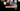# Leetcode | Solution of Transpose Matrix in JavaScript

April 5th, 2020
|

In this post, we will solve Transpose Matrix from leetcode and compute it's time and space complexities. Let's begin.

# Problem Statement

The question can be found at leetcode Transpose Matrix problem.

The problem states that we are given a two-dimensional matrix and we need to transpose it and return the new matrix.

# Solution

Transposing a matrix means, the columns of the matrix become the new rows and rows become new columns. So, if the initial matrix was of size `n*m`, the new matrix will be of `m*n`.

The approach for this one is simple. We will create an empty array. Then we will loop over the given matrix column-wise. We will keep on pushing the elements in the column to an empty array. Once we are done with one column, we will push the array of numbers to the array created.

Once we are done with all the columns, we will have a new matrix.

We have discussed the approach, I urge you to go ahead on leetcode and give it another try.If you are here, it means something went wrong in implementation or you are just too lazy. In any case, let's see a simple implementation of the above logic.
``````var transpose = function (A) {

const result = [];

for (let i = 0; i < A.length; i++) {
const col = []
for (let j = 0; j < A.length; j++) {
col.push(A[j][i]);
}
result.push(col)
}

return result
};``````

Let's look at the solution. First, we created an empty array. Next, we are looping over the matrix `A` column-wise. Notice the conditions in both loops. `A.length` will give the length of all the columns. We keep on pushing all the values in a column to an array `col`. When we are done with one column, we push the `col` array to our `result` array.

At last, we return the result array.

Here are the stats on submission

``````Status: Accepted
Runtime: 72ms
Memory: 37.5MB``````

## Time and space complexity

### Time complexity

We are looping over all the matrix elements, so time complexity would be O(n2).

### Space complexity

We are using extra space to store the new matrix. So space complexity is also constant, O(n2).

# Summary

So, we solved the transpose matrix problem and calculated the time and space complexities.

I hope you enjoyed solving this question. This is it for this one, complete source code for this post can be found on my Github Repo. Will see you in the next one.